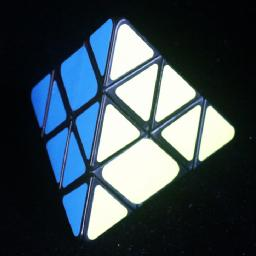# Bandaged PyraminxThis puzzle is made from a standard pyraminx by gluing an edge piece to an adjacent corner piece, and then doing the same to the edge on the opposite side of the puzzle. This is somewhat similar to how a bicube is made from a standard Rubik's cube.

This puzzle was made by Norman Diamond, and he gave one to me when he asked me to devise a solution to it.

## The number of positions:

There are three types of pieces; the tips, corner pieces and edge pieces. There are 4 corner pieces which each have 3 possible orientations. If unrestricted this would give rise to 34 combinations. On the bandaged puzzle however, the two bandaged corners can be in any orientation but one, so this leaves only (32-1)·32 orientations. The 4 unbandaged edge pieces can be in any position given the same restrictions as the normal pyraminx, i.e. they must lie in an even permutation, and the total number of flipped pieces is even. This leaves a total of (32-1)·32·4!/2·24/2 = 6,912 positions ignoring the trivial corner tips.

If you include the trivial tips then this has to be multiplied by a further 34=81.

A quick computer search gives the following result (ignoring the tips):
Depth     # Positions
01
14
212
332
476
5206
6510
71,126
81,803
91,980
10981
11165
1215
131
Total6,912

The puzzle can therefore be solved in at most 13 moves (8.1687 on average). The single antipode at distance 13 is the position with all 4 free edges flipped. In Sloane's On-Line Encyclopedia of Integer Sequences this is included as sequence A079769.

## Notation:

Hold the puzzle so that the tips on the two corner pieces which have an edge glued to them are pointing left and right, and the other two corner tips are pointing back and up. Clockwise 120 degree turns of these corners will be denoted by the letters L, R, B, U respectively. Anti-clockwise turns are denoted by an apostrophe, i.e. L', R', B', U'. The position of an edge piece can be specified by two letters, e.g. the edge in position LR is the piece lying directly between the L and R corners.

In the solved position, the edges which are glued to the L and R corner pieces lie in positions LB and RU respectively. Due to its symmetry this is true even when the puzzle is turned over so that the L and R corners are swapped.

## Solution:

Phase 1: Solve the tips.
1. Simply turn each tip so that it matches its corner piece.

Phase 2: Solve the bandaged corner pieces.

1. Find out which colour is missing from the B corner piece. The face opposite (the URL face at the front) will eventually be that colour.
2. Turn the L and R bandaged corner pieces to their correct orientations, i.e. showing the correct colour in the front face. The bandaged edges should lie at RU and LB.

Phase 3: Position the LR edge piece, in its correct orientation.

1. Find the piece that belongs in the LR position (directly between the bandaged corners). Skip this step if it is already correctly positioned and oriented.
2. If the edge lies at RB then do R', otherwise do R.
3. Turn U to bring the edge to the UB position.
4. Turn R back to its original position.
The piece belonging at LR now lies at UB.
5. If the colour of the front (ULR) face is showing on the right hand side (URB face) of the edge piece, then do RL'U'L'R'L', otherwise do RUR'. The edge should now match the adjacent L and R corner pieces.

Phase 4: Solve the unbandaged corner pieces

1. To turn the U corner, do R'UR or R'U'R so that its colours match the L corner piece.
2. To turn the B corner, do L'BL or L'B'L so that its colours match the R corner piece.

Phase 5: Position the remaining 3 edges.

1. Find the edge piece belonging at the RB position.
2. If it lies at UL, then do RURUR'UR'.
3. If it lies at UB, then do RU'RU'R'U'R'.
4. The other two edge pieces are automatically now correctly positioned.

Phase 6: Orient the 3 edges.

1. To flip the RB and UB edges, do RUL'ULU'RU'R.
2. To flip the RB and UL edges, do RUL'U'LU'RUR.
3. To flip the UL and UB edges, do LBR'BRB'LB'L.